# Understanding K-Means Clustering

Clustering algorithms are a wide range of techniques aiming to find subgroups in a dataset. Clustering models learn to assign labels to instances of the dataset: this is an unsupervised method.

The goal is to group together instances that are most similar.

Probably the simplest clustering algorithm to understand is the k-means clustering algorithm, which clusters the data into k number of clusters.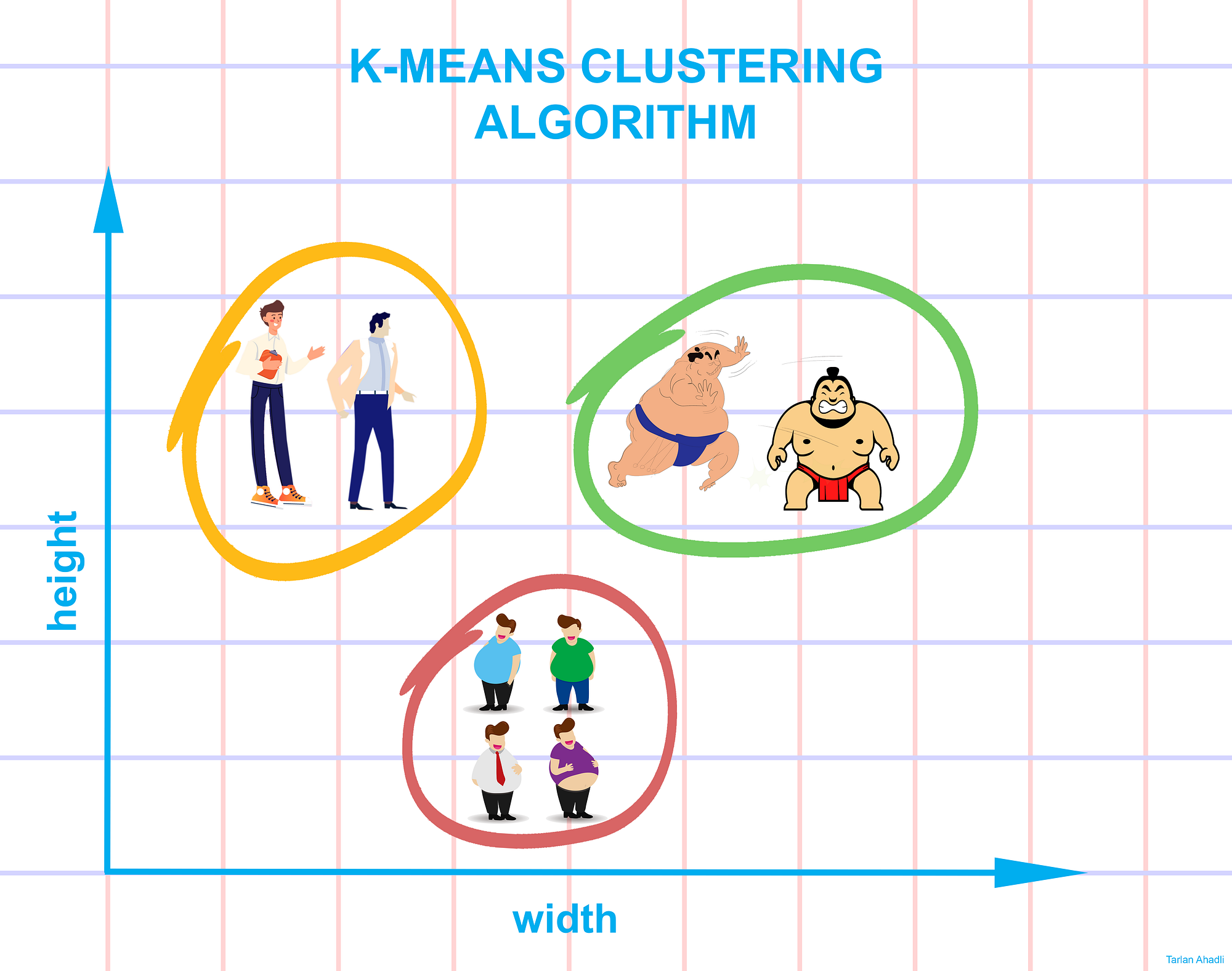## The idea behind the k-means algorithm¶

According to the k-means algorithm, an optimal clustering is a clustering in which the within-cluster variation is minimized. This within-cluster variation is usually defined as the squared Euclidean distance between the "cluster center" and each point.

A data set is well separated into k clusters when:

• The centroid is the mean of all instances within the cluster.
• Each instance is closer to its own centroid than to other centroids.

Those two assumptions are the foundations of the k-means algorithm.

Before going deeper into this algorithm, let's create a simple dataset to implement k-means.

Let's start by importing the tools of the trade:

In :
import time

# Data analysis
import numpy as np

# Visualization
import matplotlib.pyplot as plt

plt.style.use("fivethirtyeight")
%matplotlib inline

# Models and tools
from sklearn.datasets import make_blobs
from sklearn.datasets import make_moons
from sklearn.cluster import KMeans
from sklearn.metrics import pairwise_distances_argmin
from sklearn.preprocessing import StandardScaler
from sklearn.metrics import silhouette_score


For educational purposes, let's generate a small and simple dataset in two dimensions with five distinct clusters.

In :
# Identify the coordinates of the centers
blob_centers = np.array([[0.5, 2.7], [-1.5, 2.3], [1, 1.2], [-2.2, 2.8], [-2.8, 1.3]])

# Determine the standard deviation of each cluster
blob_stddev = np.array([0.4, 0.3, 0.2, 0.1, 0.1])

# Make the the clusters.
X, y = make_blobs(
n_samples=1000, centers=blob_centers, cluster_std=blob_stddev, random_state=10
)


Now let's plot the blobs that we have created to see if we can easily identify clusters in our dataset:

In :
def plot_clusters(X, clusters=None, centers=None):
# Plot the data points
plt.scatter(X[:, 0], X[:, 1], c=clusters, s=20)

# Plot the centers if they are identified
if np.any(centers):
plt.scatter(
centers[:, 0], centers[:, 1], marker="x", s=100, linewidths=10, color="r"
)

In :
plot_clusters(X)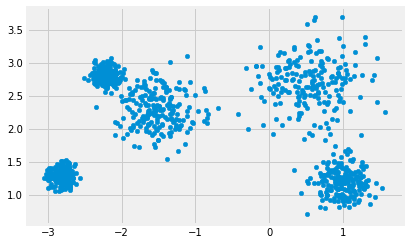Visually, it is easy to discern our five clusters.

The k-means algorithm implemented in Python's Scikit-learn library can find these clusters through its typical estimator API.

In :
%%time
kmeans = KMeans(n_clusters=5)
kmeans.fit(X)
clusters = kmeans.predict(X)

CPU times: user 60.1 ms, sys: 23.4 ms, total: 83.5 ms
Wall time: 78.8 ms


Let's visualize the results by plotting the clusters identified by the algorithm with different colors. We also plot the cluster centers, identified with a red cross.

In :
centers = kmeans.cluster_centers_

plot_clusters(X, clusters=clusters, centers=centers)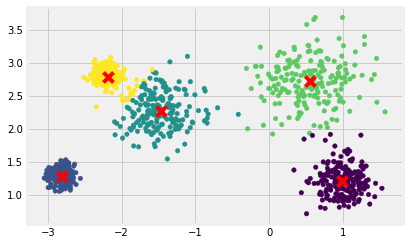The k-means algorithm easily assigned the same clusters to the ones we could visually identify.

How did it do this so quickly and easily? The number of possible combinations of clusters assignments to the data points is exponential: a brute-force approach would be very costly.

With k-means, instead, the classical approach uses an iterative approach with the Expectation-Maximization algorithm.

## K-Means Algorithm: Expectation–Maximization¶

The Expectation–Maximization algorithm involves the following iterative steps:

• Initialize centroids randomly by choosing random instances of the dataset
• Repeat the following steps until convergence (until the centroids stop moving):
• E-Step: assign each instance to the nearest centroid
• M-Step: set the centroid to the mean of its instances

The "E-step" ("Expectation step") is so-called since we update our expectation of which cluster each instance relates to.

The "M-step" ("Maximization step") is so-called since we maximize the function that determines the position of the centroids (it is done by taking the arithmetic mean of the data in each cluster).

Each iteration of the E-step and M-step will invariably lead to a better estimate of the clusters' attributes.

Let's run the K-Means algorithm for 1, 3, 5 and 10 iterations, to see how the centroids move around.

In :
# "n_init" is set to 1 to avoid a better-than-random initialization
# "algorithm" is set to "full" to get the classical EM algorithm
def kmeans_iter(X, iterations, n_clusters=5):
kmeans = KMeans(
n_clusters=n_clusters,
init="random",
n_init=1,
algorithm="full",
max_iter=iterations,
random_state=10,
)
kmeans.fit(X)
clusters = kmeans.predict(X)
centers = kmeans.cluster_centers_
return clusters, centers

In :
# Plotting how centroids and clusters move after n iterations
iter_1, centers_1 = kmeans_iter(X, 1)
iter_3, centers_3 = kmeans_iter(X, 3)
iter_5, centers_5 = kmeans_iter(X, 5)
iter_10, centers_10 = kmeans_iter(X, 10)

fig = plt.figure(figsize=[15, 12])

plt.subplot(221)
plot_clusters(X, clusters=iter_1, centers=centers_1)
plt.title("Centroids after 1 iteration")

plt.subplot(222)
plot_clusters(X, clusters=iter_3, centers=centers_3)
plt.title("Centroids after 3 iterations")

plt.subplot(223)
plot_clusters(X, clusters=iter_5, centers=centers_5)
plt.title("Centroids after 5 iterations")

plt.subplot(224)
plot_clusters(X, clusters=iter_10, centers=centers_10)
plt.title("Centroids after 10 iterations")

Out:
Text(0.5, 1.0, 'Centroids after 10 iterations')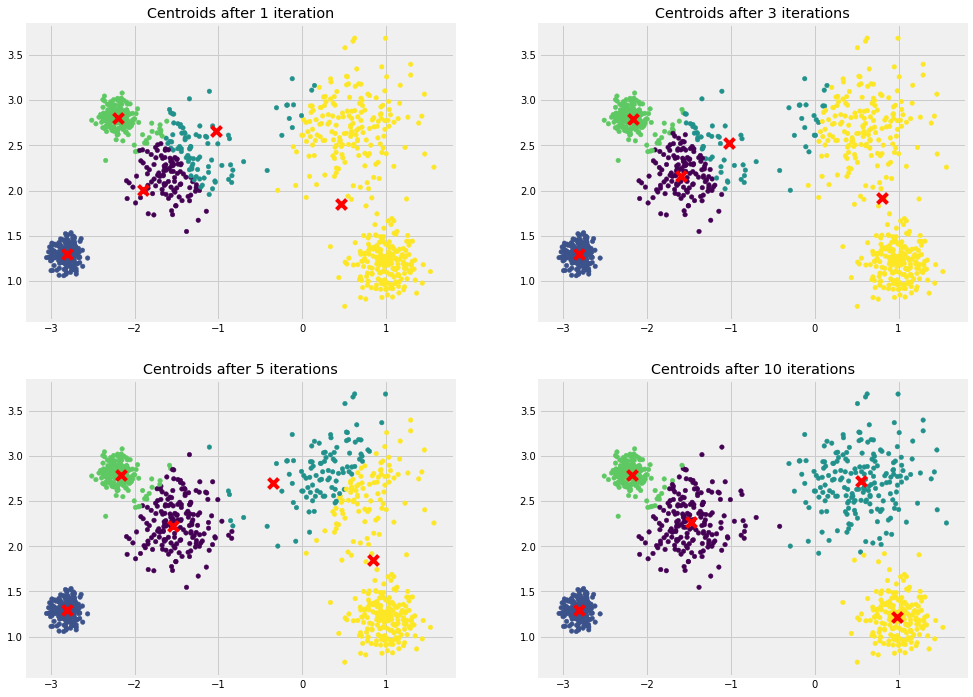## Implementing the algorithm from scratch¶

The k-means algorithm is simple enough to be implemented in a few lines:

In :
def kmeans_from_scratch(X, k_clusters, seed=42):
"""Implements the k-means algorithm from scratch"""

# Randomly choose clusters from data points
# Random number generator by shuffling data points and selecting the n first
rng = np.random.RandomState(seed)
i = rng.permutation(X.shape)[:k_clusters]
centers = X[i]

while True:
# E-step: Assign each instance to the nearest centroid
clusters = pairwise_distances_argmin(X, centers)

# M-step: set the centroïd to the mean of its instances
new_centers = np.array([X[clusters == i].mean(0) for i in range(k_clusters)])

# Break out of the loop if no more iterations
if np.all(centers == new_centers):
break

centers = new_centers

return centers, clusters


Now let's apply our homemade implementation of the algorithm and train it on our artifical dataset.

In :
centers, clusters = kmeans_from_scratch(X, 5)

plot_clusters(X, clusters=clusters, centers=centers)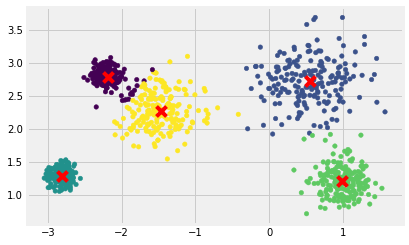## Issues with the Expectation-Maximization Algorithm¶

The Expectation-Maximization algorithm should not be use blindly, as there are a few issues you could face:

1. The optimal result is not necessarily achieved
2. The number of clusters must be picked beforehand
3. K-means is limited to linear cluster boundaries

Let's go through these issues a bit more in detail.

### 1. Optimal results and centroid initialization methods¶

In our from-scratch implementation of the k-means algorithm, the centroids are initialized randomly. Then the algorithm iterates to gradually improve centroids' positions.

However, one major problem with this approach is that if you run k-means multiple times (or with different random seeds), it can converge to different solutions.

Let's take another seed and plot the clusters again.

In :
centers, clusters = kmeans_from_scratch(X, 5, seed=100)

plot_clusters(X, clusters=clusters, centers=centers)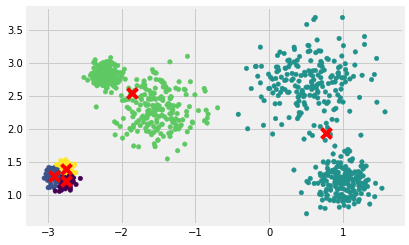The result here is clearly suboptimal. All we did was changing the random seed. The number of clusters ans the number of iterations are the same than our previous example where the model found quite good clusters. We actually even reached a poitn here where the algorithm stopped iterating: the ideal state with this random seed has been reached.

To select the best model, we need to evaluate each model's performance. Fortunately, the Scikit-learn API provides us with the so-called inertia metric, which measures the distance between each data point and its closest centroid.

The model with the lowest inertia is selected.

In :
# Inertia of sklearn's implementation of the algorithm
kmeans.inertia_

Out:
106.41004125439397

Various methods have been used to tackle this issue.

In that respect, sklearn has a parameter ("n_init") allowing the model to run n guesses with different initializations, keeping the one with the lowest inertia. It defaults to 10.

K-means++, which is an improvement to the k-means algorithm by introducing a smarter initialization, is also sklearn's default initialization method.

Other important improvements to the k-means algorithm were proposed : accelerated K-Means and mini-batch K-Means. Explanations of these improvements are out of the scope of this post.

## 2. Finding the optimal number of Clusters¶

So far, we have set the number of clusters k to 5 because it was obvious by looking at our dataset that this was the best number to pick. But more often than not it is not be so obvious and the results can be quite bad when picking the wrong number of clusters.

Indeed, setting k to 3 or 7 clusters result in obviously suboptimal clusters:

In :
# Code for k=3 and k=7
clusters_n3, centers_n3 = kmeans_iter(X, 10, n_clusters=3)
clusters_n7, centers_n7 = kmeans_iter(X, 10, n_clusters=7)

fig = plt.figure(figsize=[15, 5])

plt.subplot(121)
plot_clusters(X, clusters=clusters_n3, centers=centers_n3)
plt.title("K-means with 3 clusters")

plt.subplot(122)
plot_clusters(X, clusters=clusters_n7, centers=centers_n7)
plt.title("K-means with 7 clusters")

Out:
Text(0.5, 1.0, 'K-means with 7 clusters')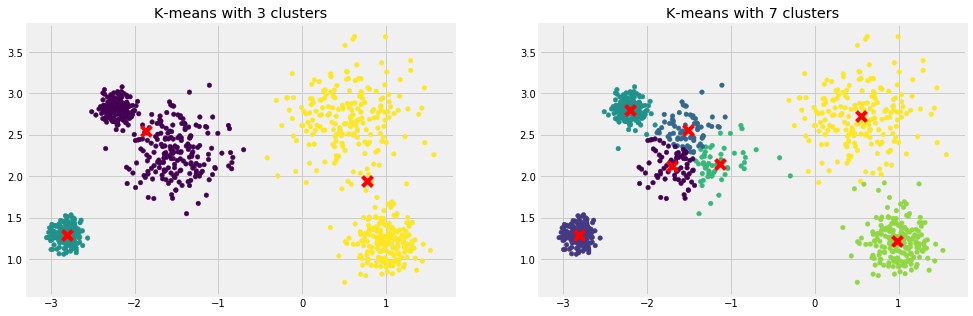We will see two methods for choosing the best value for the number of clusters:

1. Elbow method
2. Silhouette method

### Elbow Method¶

We saw a useful metric while discussing initialization methods' importance: inertia. Could we use this metric to select the right number of clusters?

Let's calculate the inertia for clusters from 2 to 10 and compare them visually.

In :
inertias = []
K = range(2,10)

for k in K:
clusterer = KMeans(n_clusters=k).fit(X)
inertias.append(clusterer.inertia_)

In :
# Lineplot using inertia for the elbow method
plt.plot(K, inertias)
plt.xlabel('K - Number of Clusters')
plt.ylabel('Inertia')
plt.title('The Elbow Method')

Out:
Text(0.5, 1.0, 'The Elbow Method')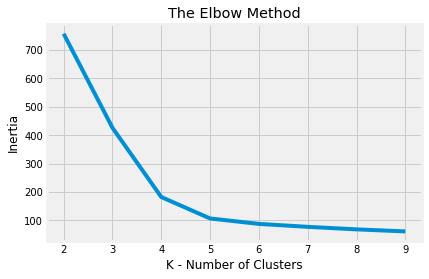When plotting the line of the elbow method, if it looks like an arm, then the point of inflection of the curve (the "elbow") is considered to be a good number of clusters to pick for the model.

Here, k = 4 or k = 5 are the two visible inflections of the arm, which corresponds to our intuition.

But the elbow method is not the most reliable for choosing k, specifically if the data is noticeably clustered. We might indeed see a curve without much inflection and it would then be uncertain of the right value for k. We might then turn ourselves towards another method for choosing k, such as computing silhouette scores.

### Silhouette Method¶

The silhouette method assesses the quality of a clustering by finding out how well each instance lies within its cluster. A high silhouette displays a good clustering.

For a technical explanation of how the silhouette method works, you can refer to this excellent blog post.

In :
silhouette_scores = []
K = range(2,10)

for k in K:
clusterer = KMeans(n_clusters=k)
preds = clusterer.fit_predict(X)
score = silhouette_score(X, preds)
silhouette_scores.append(score)

# Lineplot using silhouette score
plt.plot(K, silhouette_scores)
plt.xlabel('K - Number of Clusters')
plt.ylabel('Silhouette score')
plt.title('The Silhouette Method')

Out:
Text(0.5, 1.0, 'The Silhouette Method')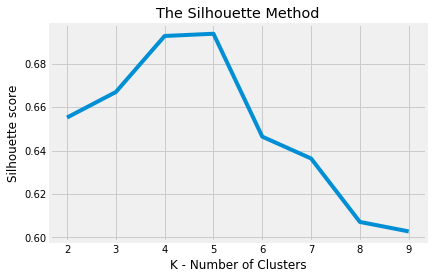As we can see, this visualization is richer than the previous one: it confirms that k = 4 or k = 5 are the best choices, and also underlines the fact that there is big difference of quality when selecting a 6th cluster. This was not visible when comparing inertias.

Hierarchical clustering is an alternative algorithm which does not require that we commit to a particular choice of clusters. You can learn more about it with the amazing Statquest and this video:

### 3. Clustering with non-linear boundaries¶

K-means make a fudamental assumption: points are ideally clustered when close to their cluster center. This assumption means that k-means is inadequate for clusters with complex shapes.

In particular, the boundaries between k-means clusters will always be linear. Consider the following data:

In :
X_moons, y_moons = make_moons(500, noise=0.05, random_state=1)
clusters, centers = kmeans_iter(X_moons, 20, n_clusters=2)

In :
plot_clusters(X_moons, clusters=clusters, centers=centers)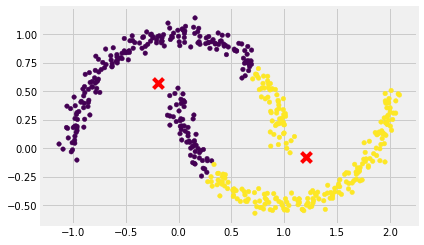As we can see, k-means' clustering decision boundaries are linear and cannot learn non-linearly distributed clusters. Depending on the data, different clustering algorithms may perform better. For example, on these types of clusters, Gaussian Mixture Models work way better.